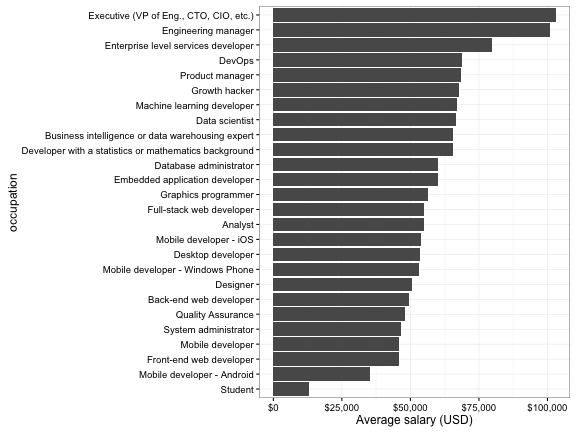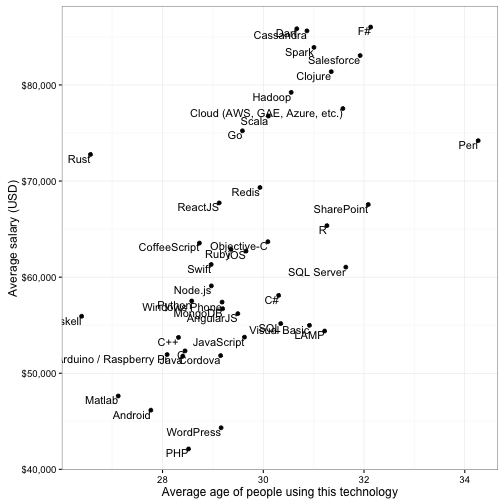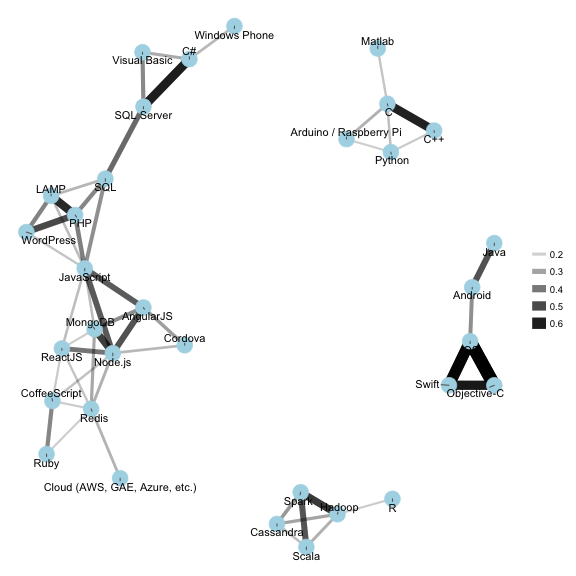# An R Package With the 2016 Developer Survey Results

### Data analysis using the R language. Here's a brand-new package that was written in R and is demonstrated using the latest Stack Overflow developer survey.

· Big Data Zone · Tutorial
Save
2.55K Views

this year, more than fifty thousand programmers answered the stack overflow 2016 developer survey , in the largest survey of professional developers in history.

last week stack overflow released the full (anonymized) results of the survey at stackoverflow.com/research . to make analysis in r even easier, today i’m also releasing the stacksurveyr package, which contains:

• the full survey results as a processed data frame ( ``` stack_survey ``` )
• a data frame with the survey’s schema, including the original text of each question ( ``` stack_schema ``` )
• a function that works easily with multiple-response questions ( ``` stack_multi ``` )

this makes it easier than ever to explore this rich dataset and answer questions about the world’s developers.

### examples: basic exploration

i’ll give a few examples of survey analyses using the dplyr package. for instance, you could discover the most common occupations of survey respondents:

``````library(stacksurveyr)
library(dplyr)

stack_survey %>%
count(occupation, sort = true)``````
``````## # a tibble: 28 x 2
##                             occupation     n
##                                  <chr> <int>
## 1             full-stack web developer 13886
## 2                                 <na>  6511
## 3               back-end web developer  6061
## 4                              student  5619
## 5                    desktop developer  3390
## 6              front-end web developer  2873
## 7                                other  2585
## 8  enterprise level services developer  1471
## 9           mobile developer - android  1462
## 10                    mobile developer  1373
## # ... with 18 more rows``````

we can also use ``` group_by ``` and ``` summarize ``` to find the highest paid (on average) occupations:

``````salary_by_occupation <- stack_survey %>%
filter(occupation != "other") %>%
group_by(occupation) %>%
summarize(average_salary = mean(salary_midpoint, na.rm = true)) %>%
arrange(desc(average_salary))

salary_by_occupation``````
``````## # a tibble: 26 x 2
##                                               occupation average_salary
##                                                    <chr>          <dbl>
## 1                 executive (vp of eng., cto, cio, etc.)      103073.93
## 2                                    engineering manager      101047.08
## 3                    enterprise level services developer       79855.62
## 4                                                 devops       68731.96
## 5                                        product manager       68598.62
## 6                                          growth hacker       67878.79
## 7                             machine learning developer       67041.80
## 8                                         data scientist       66508.75
## 9       business intelligence or data warehousing expert       65660.92
## 10 developer with a statistics or mathematics background       65625.76
## # ... with 16 more rows``````

this can be visualized in a bar plot:

``````library(ggplot2)
library(scales)

salary_by_occupation %>%
mutate(occupation = reorder(occupation, average_salary)) %>%
ggplot(aes(occupation, average_salary)) +
geom_bar(stat = "identity") +
ylab("average salary (usd)") +
scale_y_continuous(labels = dollar_format()) +
coord_flip()``````10 of the questions allow multiple responses, as can be noted in the ``` stack_schema ``` variable:

``````stack_schema %>%
filter(type == "multi")``````
``````## # a tibble: 10 x 4
##                              column  type
##                               <chr> <chr>
## 1               self_identification multi
## 2                           tech_do multi
## 3                         tech_want multi
## 4                   dev_environment multi
## 5                         education multi
## 6                     new_job_value multi
## 7  how_to_improve_interview_process multi
## 8            star_wars_vs_star_trek multi
## 9              developer_challenges multi
## 10               why_stack_overflow multi
## # ... with 2 more variables: question <chr>, description <chr>``````

in these cases, the responses are given delimited by ``` ; ``` . often, these columns are easier to work with and analyze when they are “unnested” into one user-answer pair per row. the package provides the ``` stack_multi ``` function as a shortcut for that unnesting. for example, consider the ``` tech_do ``` column (““which of the following languages or technologies have you done extensive development within the last year?”):

``stack_multi("tech_do")``
``````## # a tibble: 225,075 x 3
##            <int>   <chr>                  <chr>
## 1           4637 tech_do                    ios
## 2           4637 tech_do            objective-c
## 3          31743 tech_do                android
## 4          31743 tech_do arduino / raspberry pi
## 5          31743 tech_do              angularjs
## 6          31743 tech_do                      c
## 7          31743 tech_do                    c++
## 8          31743 tech_do                     c#
## 9          31743 tech_do              cassandra
## 10         31743 tech_do           coffeescript
## # ... with 225,065 more rows``````

using this data, we could find the most common answers:

``````stack_multi("tech_do") %>%
count(tech = answer, sort = true)``````
``````## # a tibble: 42 x 2
##          tech     n
##         <chr> <int>
## 1  javascript 27385
## 2         sql 21976
## 3        java 17942
## 4          c# 15283
## 5         php 12780
## 6      python 12282
## 7         c++  9589
## 8  sql server  9306
## 9   angularjs  8823
## 10    android  8601
## # ... with 32 more rows``````

we can join this with the ``` stack_survey ``` dataset using the ``` respondent_id ``` column. for example, we could look at the most common development technologies used by data scientists:

``````stack_survey %>%
filter(occupation == "data scientist") %>%
inner_join(stack_multi("tech_do"), by = "respondent_id") %>%
``````## # a tibble: 42 x 2
##         <chr> <int>
## 1      python   507
## 2         sql   356
## 3           r   352
## 4        java   240
## 5  javascript   207
## 6         c++   155
## 7           c   125
## 9  sql server    98
## 10      spark    97
## # ... with 32 more rows``````

or we could find out the average age and salary of people using each technology, and compare them:

``````stack_survey %>%
inner_join(stack_multi("tech_do")) %>%
summarize_each(funs(mean(., na.rm = true)), age_midpoint, salary_midpoint) %>%
ggplot(aes(age_midpoint, salary_midpoint)) +
geom_point() +
geom_text(aes(label = answer), vjust = 1, hjust = 1) +
xlab("average age of people using this technology") +
ylab("average salary (usd)") +
scale_y_continuous(labels = dollar_format())``````if we want to be a bit more adventurous, we can use the (in-development) widyr package to find correlations among technologies, and the ggraph package to display them as a network of related technologies:

``````library(widyr)
library(ggraph)
library(igraph)

set.seed(2016)

stack_multi("tech_do") %>%
filter(correlation > .15) %>%
graph_from_data_frame() %>%
ggraph(layout = "fr") +
geom_edge_link(aes(edge_alpha = correlation, edge_width = correlation)) +
geom_node_point(color = "lightblue", size = 7) +
geom_node_text(aes(label = name), repel = true) +
theme_void()``````try the data out for yourself!

Topics:
statistics, r language, stack overflow, survey

Published at DZone with permission of David Robinson, DZone MVB.

Opinions expressed by DZone contributors are their own.